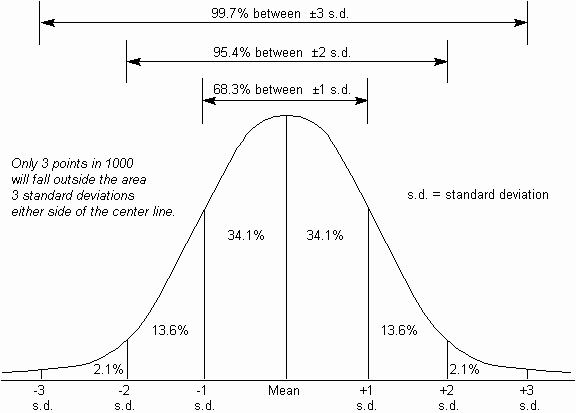The Psychology of Quality and More

The Quality Toolbook > Variation > Measuring spread

There are two main ways of measuring the degree of spread of a set of measurements: the range and the standard deviation.

## Range

The range of a set of measures is simply the difference between the largest and the smallest measurement value.

Thus, for example, if you have a set of measures (21, 22, 26, 19, 12, 24, 33) then you first find the highest measure (33) and subtract the lowest measure (12) to give the range (21).

This is easy to calculate, but there can be several problems with using it:

• Special causes of variation can cause an unrealistically wide range.
• As more measurements are made, it will tend to increase.
• It gives no indication of the data between its values.

## Standard deviation

The standard deviation is a number which is calculated using a simple mathematical trick (calculating the square root of the average of squares) to find an 'average' number for the distance of the majority of measures from the mean.

The standard deviation is of particular value when used with the Normal distribution, where known proportions of the measurements fall within one, two and three standard deviations of the mean, as below.Fig. 1. Percentages in Normal Distribution between Standard Deviations

Thus, given a set of measures, the mean and the standard deviation can be calculated, and from this can be derived the probability of future measures falling into the three bands, provided that the distribution is normal (a simple visual test for this is to draw a histogram and look for the bell shape).

For example, if the gunner has an average score of 56 per target card, with a standard deviation of 6, then, provided the distribution is normal:

• 68.3% of scores will be 56 ± 6 (= between 50 and 62)

• 95.4% of scores will be 56 ± 12 (= between 44 and 68)

• 99.7% of scores will be 56 ± 18 (= between 38 and 74)

or, breaking out the six bands:

• 2.1% of scores will be between 38 and 44

• 13.6% of scores will be between 44 and 50

• 34.1% of scores will be between 50 and 56

• 34.1% of scores will be between 56 and 62

• 13.6% of scores will be between 62 and 68

• 2.1% of scores will be between 68 and 74

• The remaining 0.3% of scores will be below 38 or above 74.### You can buy books here

And the big
paperback book